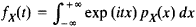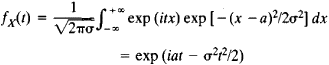# characteristic function

(redirected from Characteristic map)
Also found in: Dictionary.

## characteristic function

[‚kar·ik·tə′ris·tik ′fəŋk·shən]
(mathematics)
The function χA defined for any subset A of a set by setting χA (x) = 1 if x is in A and χA = 0 if x is not in A. Also known as indicator function.
(physics)
A function, such as the point characteristic function or the principal function, which is the integral of some property of an optical or mechanical system over time or over the path followed by the system, and whose value for a path actually followed by a system is a maximum or a minimum with respect to nearby paths with the same end points.
(statistics)
A function that uniquely defines a probability distribution; it is equal to √(2π) times the Fourier transform of the frequency function of the distribution.

## Characteristic Function

in mathematics:

(1) An eigenfunction.

(2) The characteristic, or indicator, function of a set A is a function f(x) that is defined on some set E containing A and that assumes the value f(x) = 1 if x is in A and the value f(x) = 0 if x is not in A.

(3) In probability theory, the characteristic function fx(t) of a random variable X is the mathematical expectation of the quantity exp (it X). For a random variable with probability density function PX(x), this definition yields the formulaFor example, for a random variable having a normal distribution with parameters a and σ, the characteristic function isThe characteristic function has several noteworthy properties. To every random variable X there corresponds a definite characteristic function. The probability distribution for X is uniquely determined by fX(t). When independent random variables are added, the corresponding characteristic functions are multiplied. If the concept of closeness is suitably defined, to random variables with close distributions there correspond characteristic functions that differ little from each other, and, conversely, to close characteristic functions there correspond random variables with close distributions. These properties underlie the applications of characteristic functions, particularly applications to the derivation of the limit theorems of probability theory.

A mathematical apparatus more or less equivalent to that of the characteristic function was first used by P. Laplace in 1812, but the full power of the characteristic function method was demonstrated in 1911 by A. M. Liapunov, who used the method in obtaining the theorem that bears his name.

The concept of characteristic function can be generalized to finite and infinite systems of random variables—that is, to random vectors and stochastic processes. The theory of characteristic functions has much in common with the theory of Fourier integrals.

### REFERENCES

Gnedenko, B. V. Kursteorii veroiatnostei, 5th ed. Moscow, 1969.
Prokhorov, Iu. V., and Iu. A. Rozanov. Teoriia veroiatnostei, 2nd ed. Moscow, 1973.

## characteristic function

(mathematics)
The characteristic function of set returns True if its argument is an element of the set and False otherwise.
References in periodicals archive ?
Figure 17 shows an example of such difference characteristic map.
[Q.sub.exp1] - difference characteristic map for one test configuration
These characteristic maps made of the data from the test runs enable the comparison of various test configurations.
The resulting characteristic maps describing the correlation Q - f([DELTA]T, [t.sub.i]) and the corresponding difference characteristic maps are presented in appendix.
The difference characteristic maps (see appendix) have the key importance for the efficiency analysis of different configurations of air wall to reduce heat losses through the opened doors in the bus.
The corresponding difference characteristic maps show also, that up to a doors opening time of approximately 20s the effect of air wall operation is not clearly seen to reduce heat losses.
Allocating the interpolation equations of each time interval led to characteristic maps, which depict the correlation Q = f([DELTA]T, [t.sub.i]).
It should be pointed out, however, that these investigations were carried out for the entire engine characteristic map. The relatively constant shares of the coolant pump and belt drive over the engine load can be recognized, which at this speed are shared out in a ratio of around 1:2.
The optimal settling time of the operating points for compiling the engine friction characteristic maps could be established as a result.
On the basis of the knowledge acquired, it was possible to develop an optimized measuring program for the subsequent characteristic maps and load variation investigations.
It is not hard to extend these characteristic maps to [H.sub.n](0)-modules with gradings and filtrations.

Site: Follow: Share:
Open / Close